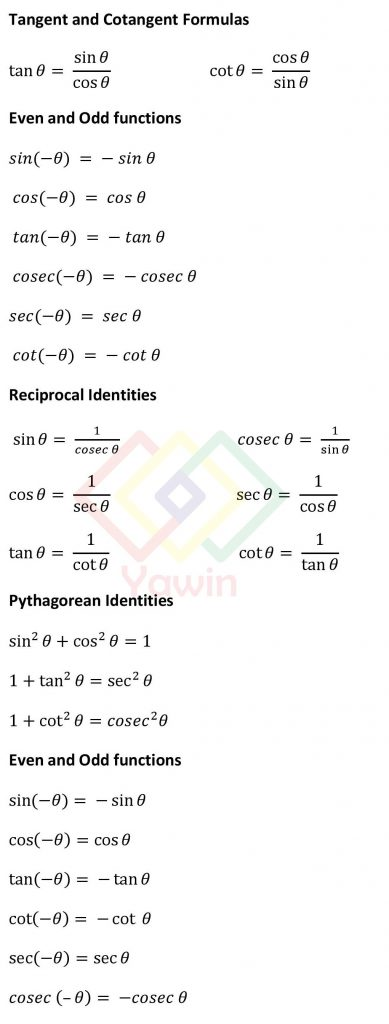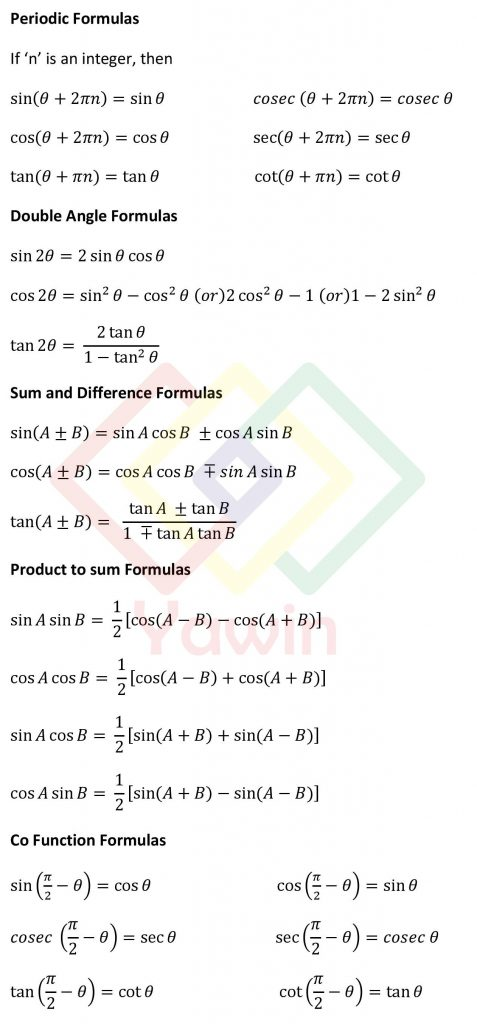Trigonometry Formula is a set of mathematical equations and relationships that are used to solve problems related to angles and sides of triangles. Trigonometry formulas are fundamental tools in the field of trigonometry and are used extensively in various disciplines such as mathematics, physics, engineering, and navigation. All Trigonometry formulas help in calculating the values of unknown angles or sides based on the given information.

Trigonometry formulas are derived from the ratios of the sides of right triangles. The three primary trigonometric functions, sine (sin), cosine (cos), and tangent (tan), along with their reciprocal functions, cosecant (csc), secant (sec), and cotangent (cot), form the basis of these formulas. These functions are defined based on the ratios of the lengths of specific sides of a right triangle.

The following is a list of all Trigonometry Formulas.## Trigonometry Formula Topics

Trigonometry Formula and Identities

Trigonometry Tangent and Cotangent Formulas

Trigonometry Even and Odd functions

Trigonometry Reciprocal Identities

Trigonometry Pythagorean Identities

Trigonometry Pythagorean Even and Odd functions

Trigonometry Periodic Formulas

Trigonometry Double Angle Formulas

Trigonometry Sum and Difference Formulas

Trigonometry Product to sum Formulas

Trigonometry Co Function Formulas